Journal of Measurements in Engineering

Published: 29 September 2022

# Effect of warp and weft uniaxial tension on the permeability of sand covered with geotextile

Xiaolei Man1
Hongge Li2
Hao Xiao3
Hanyue Wang4
Mengyu Rong5
1, 3, 4, 5College of Civil and Architecture Engineering, Chuzhou University, Chuzhou, 239000, China
2Weihai Nanhai New Area Housing, Transportation and Emergency Management Bureau, Weihai, 264200, China
Corresponding Author:
Xiaolei Man
Editor's Pick
Views 58

#### Abstract

The permeability of sand covered with geotextile is affected by the permeability of geotextile, which is related to the tensile state of the geotextile. Considering the weaving mode of geotextile, the effects of warp tension and weft tension on the permeability of sand covered with geotextile were studied by experiment. Four different specifications of geotextiles were selected for warp and weft tension respectively. The changes of permeability parameters of sand covered with geotextile under non tension, warp and weft tension were measured by vertical permeability instrument, and the effects of warp and weft tension on permeability parameters such as seepage velocity, sand loss and gradient ratio were analyzed. The test results show that the water permeability and anti silting performance of the geotextile increase with the increase of tensile strain, and the soil conservation performance decreases with the tensile strain increasing. Meanwhile, the relationship between permeability and warp tensile strain is not monotonic. When the warp tensile strain 3%, the water permeability and anti silting performance of geotextile are the weakest, and the soil conservation performance is the strongest.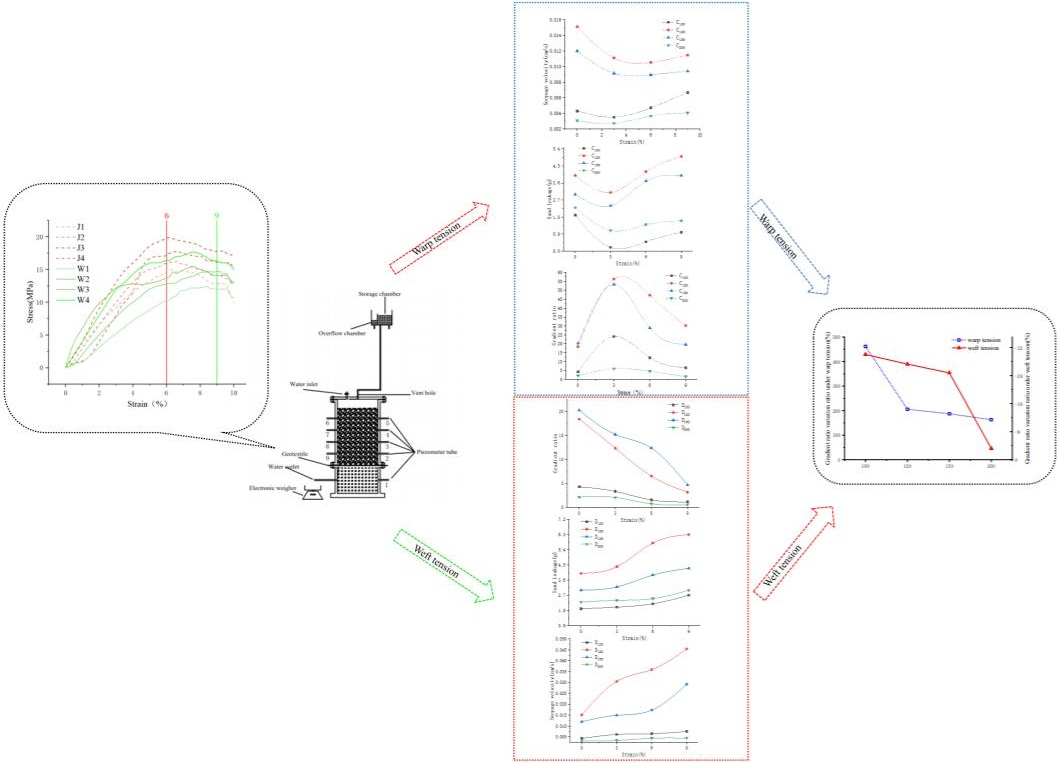#### Highlights

• The effects of warp tension and weft tension on the permeability of sand covered with geotextile were considered.
• Through sand covered with geotextile permeability tests with four different geotextiles under warp and weft tension, the influence of different tensile strains on the permeability of geotextiles covered with sand is analyzed.
• The permeability of sand covered with geotextile under different tensile strains was studied. The effects of seepage velocity, sand leakage and gradient ratio with tensile strain on permeability are analyzed. And under the same strain, the geotextiles of the same specification were stretched by warp tension and weft tension respectively.

## 1. Introduction

Geotubes are widely used in the construction of many kinds of infrastructures, such as the reservoirs in estuarine area, the channels in deep water, and the other offshore traffic projects [1-3]. Geotubes are heterogeneous systems composed of geotextiles and filling sand. Therefore, the research on the seepage characteristics of geotubes is based on the research on the permeability of sand covered with geotextiles.

Zhou B et al. obtained the influence law of hydraulic gradient on the inverted filtration performance of soil non-woven geotextile system by conducting different hydraulic gradient tests on the inverted filtration system composed of five selected non-woven geotextiles and soil . Yi J. R. et al. conducted gradient ratio tests on the system composed of non-woven fabric, high-strength woven cloth, ordinary woven cloth and sludge under different hydraulic gradients, and obtained the influence law of different hydraulic gradients on permeability . The effects of geotextile pore size and particle size on soil conservation performance, dewatering rate and dewatering degree of geotube were studied through orthogonal test . Duan G. J. et al. used filter screen instead of geotextile to study the reverse filtration and soil conservation performance of geotextile, and successively studied the reverse filtration and soil conservation effect of single layer and multi-layer filter screen under different aperture ratio. The results show that the arching of particles near the pores is the reason for the soil retention of the filter layer. The stability of particle arch and the soil retention of geotextile decrease with the increase of pore diameter ratio . The multi-functional permeability test device independently developed by Wu G. is used to study the permeability characteristics of pure sand and woven geotextile covered with sand. By comparing the permeability characteristics of pure soil and geotextile covered soil, it is analyzed that geotextile has a certain inhibitory effect on soil seepage . However, when the pore ratio of silt is large and the content of fine sand is large, the permeability coefficient under sand is greater than that of pure silt. Man X. L. et al. studied the influence of sand particle size on the permeability characteristics of pure sand and sand covered with geotextiles by constant head tests, and put forward the calculation formulas of their permeability coefficients .

However, in practical engineering, geotubes are often subjected to tensile forces of different sizes and directions, resulting in changes in its permeability characteristics. Therefore, many studies on the permeability characteristics of geotextile under tensile state have also been carried out.

She W. et al. studied the silting performance of geotextile with gradient ratio tests. The test results show that the silting degree of the system increases with the increase of tensile strain of geotextile. Tang L. et al. conducted gradient ratio test on the inverted filtration system composed of discontinuous soil and geotextiles stretched to different strains. The test results show that the unidirectional tension leads to the increase of the equivalent pore diameter of the textile and the decrease of the equivalent pore diameter of the non-woven, resulting in different changes in the filtration properties of the two fabrics. Hong Y. S. et al. studied the change of permeability coefficient of geotextile under different types of loads such as continuous load, impulse load and composite load. The test results show that the overall permeability coefficient of geotextile system increases with the increase of load. Fourier A. B. et al. carried out uniaxial tensile and biaxial tensile tests on geotextiles with different thickness. The test results show that the equivalent pore size of geotextiles will change with the change of tensile force. Wu C. S. et al. conducted water permeability test and gradient ratio test on geotextile with unidirectional tension along the weft direction. The test results show that the equivalent pore diameter, pore and permeability coefficient of geotextile will increase when in tension .

In the weaving mode of woven geotextile, the warp wire is bent, and the weft wire remains straight. When under tension, the warp first becomes straight and then deforms, while the weft directly deforms. Therefore, under the same tension, the influence of unidirectional tension in different directions on the permeability of geotextiles is different.

Through the hanging bag test, Man X. L. et al. found that the weaving mode of geotextile led to the influence of the sewing direction of the hanging bag on the dewatering performance of the hanging bag, and studied the dewatering performance of the hanging bag with different sewing modes [15, 16].

Considering the weaving mode of geotextile, the effects of warp tension and weft tension on the permeability of sand covered with geotextile were studied by experiment in this research.

## 2. Test materials and test process

### 2.1. Test materials

The permeability test of geotextile and sand covered with geotextile system under warp and weft tension was carried out with vertical permeability meter. As shown in Fig. 1, the test device consists of a water storage chamber, a middle chamber and a bottom chamber. The upper water storage chamber is connected with the water inlet of the middle chamber to provide it with constant head. The middle chamber and the bottom chamber are connected by a flange, and the stretched geotextile is fixed in the middle of the flange. Three piezometer tubes are set on the side wall of the middle chamber to calculate the permeability parameters. The bottom chamber can collect sand particles passing through the geotextile. The water outlet on the side wall of the bottom chamber can measure the water output.

The soil with good grading has good inverted filtering performance, which is not easy to highlight the inverted filtering effect of geotextile. In these tests, discontinuous graded sand is selected to test the inverted filtration performance of tensile geotextile. The particle grading information of the test sand is shown in Table 1.

Fig. 1Test equipment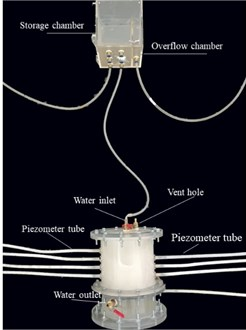a) Sketch map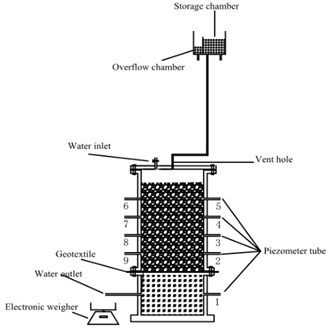b) Physical map

Table 1Information of filling sand

 Fine content Characteristic particle size / mm Nonuniformity coefficient${C}_{u}$ Curvature coefficient${C}_{c}$ ${d}_{10}$ ${d}_{30}$ ${d}_{60}$ 10 % 0.075 0.43 0.6 8.0 4.11

Four kinds of geotextiles with unit mass of 100 g, 120 g, 150 g and 200 g were selected for warp and weft tensile test. The meridional tensile test numbers were J1, J2, J3 and J4; The weft tensile test numbers were W5, W6, W7 and W8. The results of 8 groups of tensile tests are shown in Fig. 2.

Fig. 2Stress-strain curve of geotextile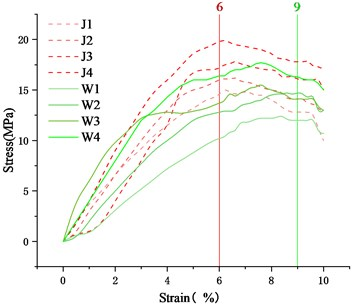As shown in Fig. 2, when the weft tensile strain reached 6 %, the tensile stress of the geotextile under weft tension reached the maximum, while when the warp tensile strain was 9 %, the warp tensile stress reached the maximum. Moreover, when the tensile strain reached 10%, each geotextile gradually failed as shown in Fig. 3. Therefore, tensile strains of 3 %, 6 % and 9 % were selected as test conditions.

Furthermore, it is found that the influence of warp and weft uniaxial tensile on the equivalent aperture of geotextile is different in the warp and weft uniaxial tensile test of geotextile. Within a certain tension range, the warp tension makes the equivalent aperture of geotextile smaller, while the weft tension makes the equivalent aperture of geotextile larger. The geotextiles under warp and weft tension are shown in Fig. 4 and Fig. 5 respectively.

Fig. 3Tensile failure state of geotextile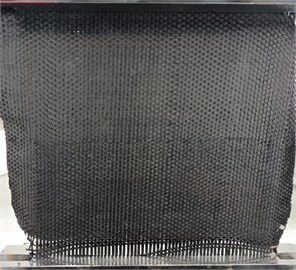Fig. 4Geotextile under warp tension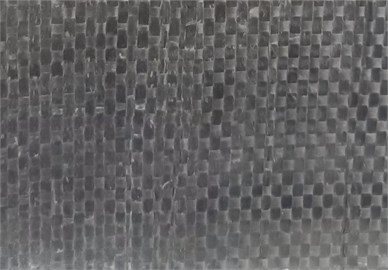Fig. 5Geotextile under weft tension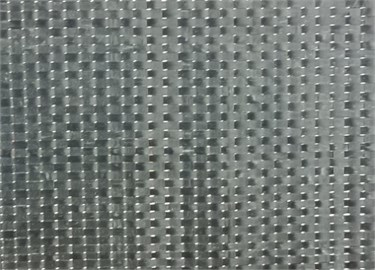### 2.2.1. Permeability test

The water permeability of geotextile under warp and weft uniaxial tension is studied with water permeability tests. In the tests, all specifications of geotextiles were stretched in warp and weft directions until the strain reached 0 % (unstressed), 3 %, 6 % and 9 %. The stretched geotextile was fixed by the middle chamber and bottom chamber of the vertical permeameter. Then the water storage chamber was connected to the water inlet of the middle chamber. The weight of water from the water outlet of the bottom chamber was weighed, and the water flow velocity through the geotextile was calculated. The geotextile specification, tensile direction and tensile strain were taken as variables in orthogonal tests, and the experimental grouping is shown in Table 2.

Table 2Permeability test conditions

 Condition group number Test group number Geotextile specification Tensile direction A A100 100 g Warp A120 120 g A150 150 g A200 200 g B B100 100 g Weft B120 120 g B150 150 g B200 200 g

The same as the permeability tests, all specifications of geotextiles were stretched in warp and weft directions until the strain reached 0 % (unstressed), 3 %, 6 % and 9 % and then fixed by the middle chamber and bottom chamber of the vertical permeameter. Before the water storage chamber was connected to the water inlet of the intermediate chamber, the sand prepared according to the certain gradation was loaded into the middle chamber. The sand needs to be soaked for 12 hours to ensure that all the air in the vertical permeameter is discharged. Then the water outlet of the bottom chamber was opened, and the water seepage and water pressure were measured. And after the tests, the sand in the bottom chamber was dried and weighed. the water storage chamber was connected to the water inlet of the middle chamber. Based on these test data, three permeability characteristic parameters of seepage velocity, sand loss and gradient ratio were calculated. The geotextile specification, tensile direction and tensile strain were taken as variables in orthogonal tests, and the experimental grouping is shown in Table 3.

 Condition group number Test group number Geotextile specification Tensile direction C C100 100 g/m2 Warp C120 120 g/m2 C150 150 g/m2 C200 200 g/m2 D D100 100 g/m2 Weft D120 120 g/m2 D150 150 g/m2 D200 200 g/m2

## 3. Test results analysis

### 3.1. Permeability test results

The effect of warp tension on the flow velocity drawing through the geotextile is shown in Fig. 6, while the effect of weft tension is shown in Fig. 7.

Fig. 6Variation of flow velocity with warp tension strain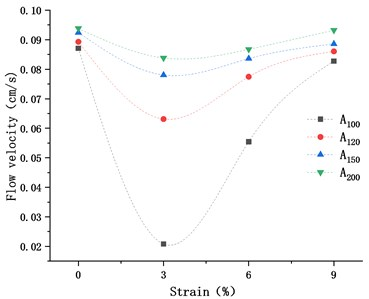Fig. 7Variation of flow velocity with weft tension strain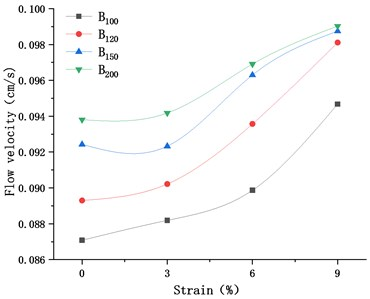As shown in Fig. 6, the flow velocity drawing through the geotextile decreases first and then increases with the increase of warp tensile strain. When the warp tensile strain is 3 %, the flow velocity reaches the minimum. It shows that the pore of geotextile decreases first and then increases with the increase of warp tensile strain, and the change of pore is mainly caused by the straightening of warp before the warp strain reaches 3 %. In Fig. 7, when subjected to weft tension, the flow velocity increases continuously with the weft tensile strain increasing.

Fig. 8 shows the change comparison of flow velocity drawing through the geotextile under the warp and weft tensile strain of 3 %. Under the warp tension, the relative variation ratio maximum of flow velocity is close to 80 %, while under the weft tension, the relative variation ratio of flow velocity cannot exceed 5 %. In addition, with the increase of geotextile thickness, the effects of warp tensile strain and weft tensile strain on geotextile flow velocity both decrease gradually.

Fig. 8Relative variation ratio of flow velocity of various geotextiles with tension strain at 3 %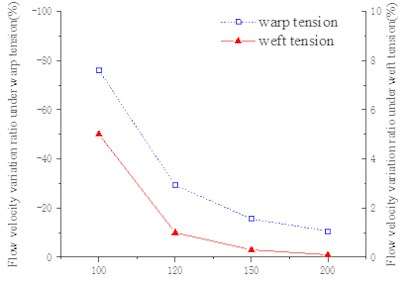For the same kind of geotextile, the comparisons of the effects of warp tension and weft tension with the same strain on the flow velocity drawing through the geotextile are shown in Fig. 9.

Fig. 9Comparison of flow velocity of geotextiles under warp and weft tension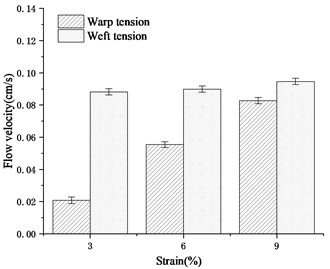a) A100 & B100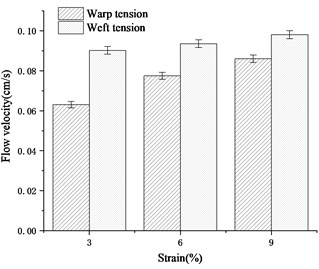b) A120 & B120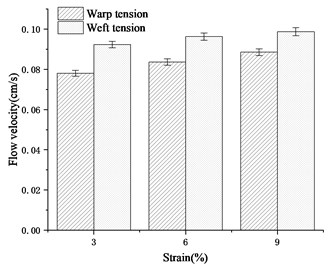c)A150 & B150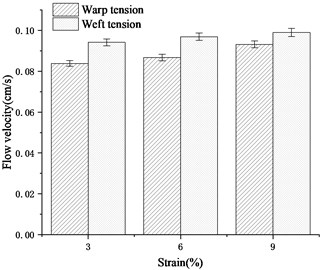d) A200 & B200

As shown in Fig. 9, under the same strain, the flow velocity drawing through the same kind of geotextile stretched along the weft direction is much greater than that of the geotextile stretched in the warp direction.

Fig. 10 shows the flow velocity relative difference value of various geotextiles under warp and weft tension. With the increase of geotextile thickness, the difference of the influence of warp tension and weft tension on the flow velocity of geotextile decreases gradually.

Fig. 10Flow velocity relative difference value of geotextiles under warp and weft tension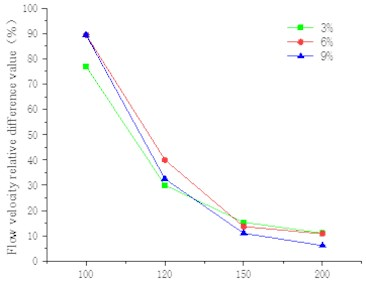### 3.2.1. Seepage velocity and sand loss

Fig. 11 and Fig. 12 show the variation of seepage velocity and sand loss with tensile strain.

Fig. 11Variation of seepage velocity with warp and weft tension strain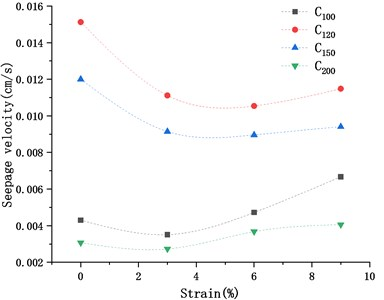a) Warp tension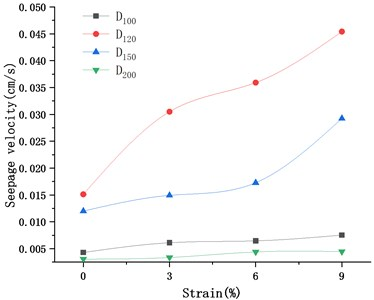b) Weft tension

Fig. 12Variation of sand loss with warp and weft tension strain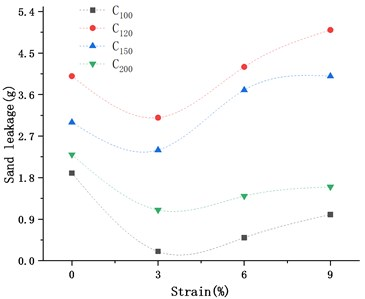a) Warp tension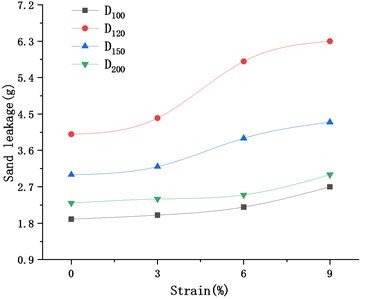b) Weft tension

As shown in the two figures, when the geotextile subjected to meridional tension, the variation of the seepage velocity and the variation of the sand loss of the sand covered with geotextile are consistent, both of which first decreases and increases with the increase of warp tensile strain. However, when the geotextile subjected to weft tension, the seepage velocity and sand loss of the sand covered with geotextile will increase with the tensile strain increasing.

The gradient ratio gr of this test is calculated as follows:

1
$GR=\frac{\frac{{H}_{1-2}}{25+\theta }}{\frac{{H}_{2-3}}{25}},$

where, ${H}_{1-2}$ is the pressure difference between piezometer tube 1 and piezometer tube 2, ${H}_{2-3}$ is the pressure difference between piezometer tube 2 and piezometer tube 3, and $\theta$ is the thickness of the geotextile.

Fig. 13Variation of gradient ratio with time in Test A100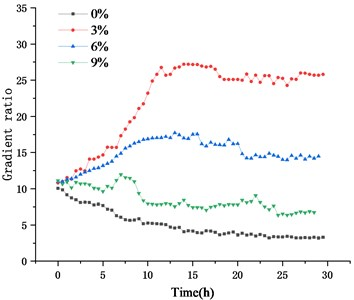Fig. 13 shows the variation of gradient ratio with time in Test A100. Similar to the law of this set of data, the gradient ratio of Geotextiles in other test groups would reach stability after the test lasting for 30 hours. Therefore, the gradient ratios at 30 hours after the start of the tests were selected as the test results.

The effect of warp tension on the gradient ratio of geotextile is shown in Fig. 14, while the effect of weft tension is shown in Fig. 15.

Fig. 14Variation of gradient ratio with warp tension strain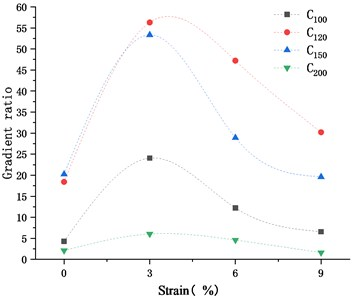Fig. 15Variation of gradient ratio with Weft tension strain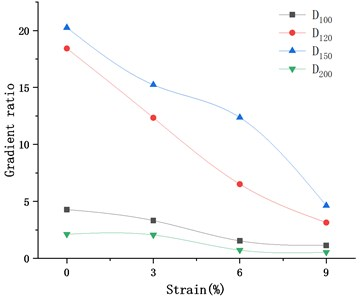As shown in Fig. 14, the gradient ratio of geotextile increases first and then decreases with the increase of warp tensile strain. When the warp tensile strain is 3 %, the gradient ratio of geotextile reaches the maximum. It shows that the reduction of equivalent pore size of geotextile inhibits the loss of fine particles and is conducive to the formation of inverted filter. Meanwhile, when the geotextile subjected to weft tension, the equivalent pore size of the geotextile gradually increases, resulting in the continuous decrease of the reverse filtration performance of the geotextile, as shown in Fig. 15.

Fig. 16Relative variation ratio of gradient ratio of various geotextiles with tension strain at 3 %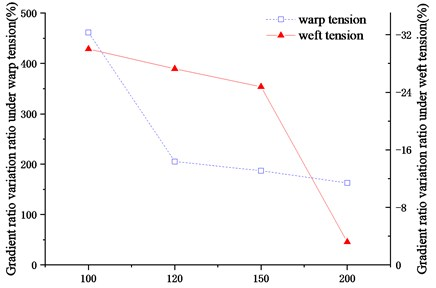Fig. 17Comparison of gradient ratio of geotextiles under warp and weft tension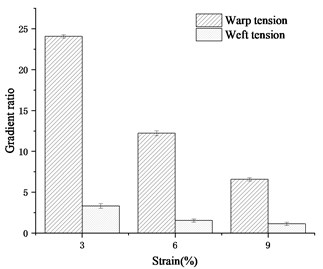a) A100 & B100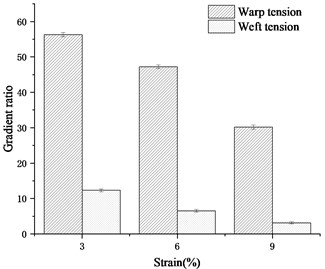b) A120 & B120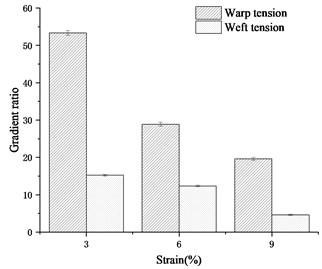c)A150 & B150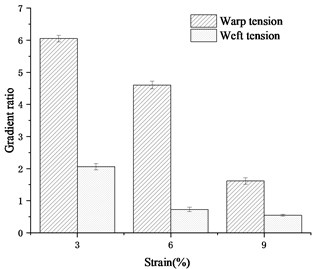d) A200 & B200

Fig. 16 shows the change comparison of gradient ratio of geotextile under the warp and weft tensile strain of 3 %. Under the warp tension, the relative variation ratio maximum of gradient ratio of geotextile is close to 500 %, while under the weft tension, the relative variation ratio of gradient ratio of geotextile is only about 30 %. Moreover, with the increase of geotextile thickness, the effects of warp tensile strain and weft tensile strain on geotextile gradient ratio both decrease gradually.

For the same kind of geotextile, the comparisons of the effects of warp tension and weft tension with the same strain on the gradient ratio of geotextile are shown in Fig. 17.

As shown in Fig. 17, under the same strain, the gradient ratio of the same kind of geotextile stretched along the warp direction is much greater than that of the geotextile stretched in the weft direction.

Fig. 18 shows the gradient ratio relative difference value of various geotextiles under warp and weft tension. With the increase of geotextile thickness, the difference of the influence of warp tension and weft tension on the gradient ratio of geotextile decreases gradually.

Fig. 18Gradient ratio relative difference value of geotextiles under warp and weft tension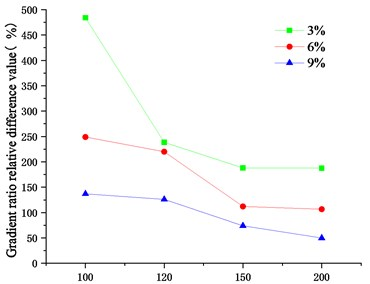## 4. Conclusions

1) When the geotextile subjected to weft tension, the water permeability and anti silting performance of the geotextile increase with the increase of tensile strain, and the soil conservation performance decreases with the tensile strain increasing.

2) When the warp tensile strain 3 %, the water permeability and anti silting performance of geotextile are the weakest, and the soil conservation performance is the strongest. When the warp tensile strain is less than 3 %, the water permeability and anti silting performance of geotextile both decrease with the increase of tensile strain, and the soil conservation performance increases with the tensile strain increasing. When the meridional tensile strain exceeds 3 %, the influence law is opposite.

3) The sensitivity of the influence of warp tension and weft tension on the permeability of geotextiles is different. Under the same strain, the influence of warp tension on the permeability of geotextile is great, while the influence of weft tension is small. But the influence difference between warp tension and weft tension on the permeability of geotextile gradually decreases with the tensile strain increasing.

4) The difference of the influence of warp tension and weft tension on the permeability of geotextile decreases with the increase of the thickness of geotextile.

#### Acknowledgements

This research was funded by the Natural Science Foundation of China (51978472) and the Key Research Project of Natural Science in Colleges and Universities of Anhui Province (KJ2021A1099).